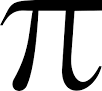# Radiometric conversion of sentinel 2 images

The process of converting values to TOA Reflectance (L1C product) into TOA spectral radiance is described on the page:

http://s2tbx.telespazio-vega.de/sen2three/html/r2rusage.html?highlight=quantification

rToa = (float)(DN_L1C_band / QUANTIFICATION_VALUE);

L = (rToa * e0__SOLAR_IRRADIANCE_For_band * cos(Z__Sun_Angles_Grid_Zenith_Values)) / (PI * U__earth_sun_distance_correction_factor);

The desired values can be found in the xml file of the L1C product.

However, there is one weird thing that I encountered during processing.
It is associated with the presence data whose values exceed QUANTIFICATION_VALUE.
For example, data for 2016 QUANTIFICATION_VALUE in the scene with the snow coverage is 10,000!
But, most of the values in this scene exceeds QUANTIFICATION_VALUE. While my question is how to be in this case – the answer is no.

2 Likes

## Direi che Igor è molto preciso.

Angelo Palombo
Area della Ricerca CNR di Tor Vergata
Via del fosso del cavaliere 100
00133 Roma - Italy
Tel: +39 0645488022
Fax: +39 0649934211
Skype palombo.angelo

Igor forum@step-email.net ha scritto:

Hi Igor, sorry for the mail sent you. The mail was sent you for an
arror, it was for share with collegues your precise responce.

## Reguard Angelo

Angelo Palombo
Area della Ricerca CNR di Tor Vergata
Via del fosso del cavaliere 100
00133 Roma - Italy
Tel: +39 0645488022
Fax: +39 0649934211
Skype palombo.angelo

Igor forum@step-email.net ha scritto:

Dear all,
first of all, for some images (recently acquired) there are only 12 values for physical gains instead of 13 and I haven’t found yet any explanation about their meaning. Are they useful in some way for reflectance to radiance conversion? Which bands do they refer to?

A second question concerning the expression for conversion:
in this topic Distance of Earth-Sun roTOA is both multiplied and divided by U factor in 2 different posts and in this own topic is multiplied.
Which is the correct expression?
L = (UrToaESUNcos(theta))/PI
or
L = (rToa
ESUNcos(theta))/(PIU)
thanks a lot
ilaria

Hi Ilaria,
I’m using is the following equation:
cos(SZA) * u / (2*pi)

similar to the your first equation however divided by 2*pi and not pi.

Angelo

It seems to me that this formula is correct:
L = (UrToaESUN*cos(theta))/PI;
Watch the discussion here:

Detailed description here:
https://sentinel.esa.int/web/sentinel/technical-guides/sentinel-2-msi/level-1c/algorithm

Correct formula:
L = (U__earth_sun_distance_correction_factor* rToa * e0__SOLAR_IRRADIANCE_For_band * cos(Z__Sun_Angles_Grid_Zenith_Values)) / PI;

Detailed:

Hello all,

Just noticed this forum.

This has been working for me when converting my L1C rTOA to LTOA (top of atmosphere radiance).

LTOA = (rTOA * ESUN * cos(SunZe_grid)) / (pi * (d^2))

where the earth sun distance, d, at any time, in theory, can be calculated by just knowing the Julian Day the image was taken.

To find the earth sun distance…

First calculate the Julian day:

A = round(year ./ 100)

B = 2 - A + round(A / 4)

JD = round(365.25 * (year + 4716)) + round(30.6001 * (month + 1)) + day + (UT / 24.0) + B - 1524.5

(round just means the output should be in integer format)

Now find the earth sun distance!

D = JD - 2451545.0;
g = 357.529 + 0.98560028 .* D;
d = 1.00014 - 0.01671 .* cosd(g) - 0.00014 .* cosd(2 .* g);

Plug d into the first equation I provided above. Hope this helps.

Ben

What is ‘PI’ in the equation?

Hello,
I think its a count 3.14159…Correct me if I am in mistake

Regards

Thank you very much kiryl23

Hi All,
I am bit confused on the radiometric convertion. Is this on for reflectance value? What is the different with sen2cor approach?
Thanks

Hi Ben,
For this band math, should it be done before using the sen2cor or after?
In my research, I will using S2 and Landsat-8 for data fusion.
Regards

A lot has changed since I posted that answer. Maybe I can make your life easier. What reflectance product are you trying to achieve and maybe I can answer your question more thoroughly?

Ben

Not sure if you got my email. Anyway, Sentinel-2A images are in JP2000 format. When importing in a GIS or matrix laboratory, divide each band by the scaling factor (10,000) to retrieve TOA reflectance (rTOA as I have posted in the formula above). Use rTOA to retrieve TOA Radiance in the equation above. Hope this helps. Sorry it took me a while to reply.

Ben

Hi Ben,
Thank you for the information. Actually, I am bit confused on the radiometric convertion and sen2cor. Since I need the reflectance value for the fusion between S2 and L8. I wonder which is more practical to be used ( radiometric convertion and sen2cor) and whether it is the same process or can be integrated.
For example L8, I have already process up to atmospheric correction and convert it to reflectance value using band math. Is it the same process for S2?
Regards